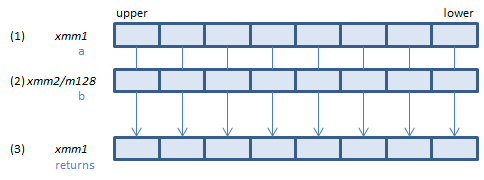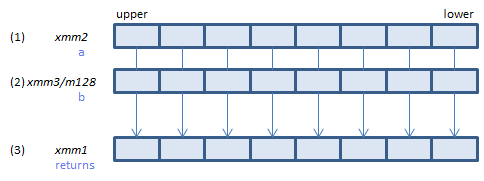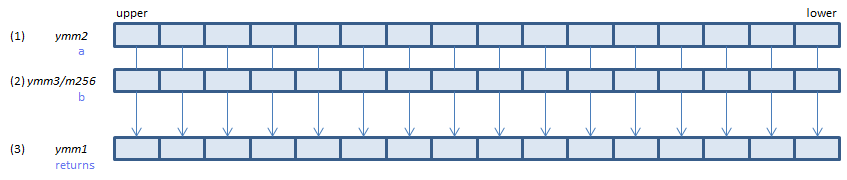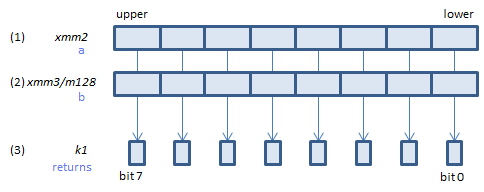﻿ pcmpeqw

## PCMPEQW - Packed CoMPare EQual Word

PCMPEQW xmm1, xmm2/m128    (S2
__m128i _mm_cmpeq_epi16(__m128i a, __m128i b)For each WORD, if (1) == (2) set 1, else set 0, to all bits in the corresponding WORD of (3).
VPCMPEQW xmm1, xmm2, xmm3/m128    (V1
__m128i _mm_cmpeq_epi16(__m128i a, __m128i b)For each WORD, if (1) == (2) set 1, else set 0, to all bits in the corresponding WORD of (3).
VPCMPEQW ymm1, ymm2, ymm3/m256    (V2
__m256i _mm256_cmpeq_epi16(__m256i a, __m256i b)For each WORD, if (1) == (2) set 1, else set 0, to all bits in the corresponding WORD of (3).
VPCMPEQW k1{k2}, xmm2, xmm3/m128    (V5+BW+VLFor each WORD, if (1) == (2) set 1, else set 0, to the corresponding bit of (3).
If k2 bit is 0, the comparison is not done and the corresponding bit of (3) is set to zero. Upper bits of (3) are zero cleared.
VPCMPEQW k1{k2}, ymm2, ymm3/m256    (V5+BW+VL